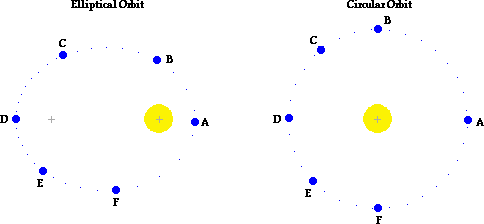## Orbital Motion

### Purpose:

The purpose of this activity is to investigate the nature of an elliptical orbit of a planet or other satellite about the Sun or some central body.

### Procedure and Questions:

1. Navigate to the Orbital Motion page and experiment with the on-screen buttons in order to gain familiarity with the control of the animation. The eccentricity of the elliptical orbit can be varied by using the sliders or the buttons. A trace of the object's motion can be turned on, turned off and erased. The vector nature of velocity and force can be depicted on the screen. The animation can be started, paused, continued or rewound.

After gaining familiarity with the program, use it to answer the following questions:

2. Velocity is a vector quantity which has both magnitude and direction. Using complete sentences, describe the object's velocity during the course of the elliptical orbit. Comment on both the magnitude and the direction.

3. Net force is also a vector quantity which has both magnitude and direction. Using complete sentences, describe the net force acting upon the object during the course of the elliptical orbit. Comment on both the magnitude and the direction.

4. Describe how an alteration in eccentricity of the orbit effects the elliptical and circular nature of it.

5. What eccentricity value results in a circular orbit? ___________

6. A circular orbit and a highly elliptical orbit are shown in the diagram below. Draw and label the net force (F) and velocity (v) vectors for each of the six marked positions for a counter-clockwise orbit. Draw the vectors in the appropriate direction and of the proper magnitude (relatively speaking).7. Complete the following sentences by filling in the blanks:

For elliptical obits: the direction of the velocity of the satellite is ___________________ (always, seldom, never) perpendicular to the net force acting upon the satellite.

For circular obits: the direction of the velocity of the satellite is ___________________ (always, seldom, never) perpendicular to the net force acting upon the satellite.

8. Fill in the blanks in the following paragraph:

If a satellite is orbiting the Earth in elliptical motion, then it will move ___________ (slowest, fastest) when its closest to the Earth. While moving towards the Earth (along the path from C to D) there is a component of force in the ________ (same, opposite) direction as the motion; this causes the satellite to ___________ (slow down, speed up). While moving away from the Earth (along the path from D to A) there is a component of force in the ________ (same, opposite) direction as the motion; this causes the satellite to ___________ (slow down, speed up).

### Summary Statement:

Summarize your findings from this lab.